﻿ 应用近场速度记录快速估算川滇地区中强地震强度文章快速检索
 地震地磁观测与研究2020, Vol. 41Issue (3): 31-36  DOI: 10.3969/j.issn.1003-3246.2020.03.0040

### 引用本文LIANG Shanshan, LIANG Jianhong, ZOU Liye, et al. Rapid determination of equivalent moment magnitude of moderately strong earthquakes in the Sichuan-Yunnan region using near-field velocity records[J]. Seismological and Geomagnetic Observation and Research, 2020, 41(3): 31-36. DOI: 10.3969/j.issn.1003-3246.2020.03.004.### 文章历史

1. 中国北京 100045 中国地震台网中心;
2. 中国北京 100081 国家海洋环境预报中心

Rapid determination of equivalent moment magnitude of moderately strong earthquakes in the Sichuan-Yunnan region using near-field velocity records
LIANG Shanshan 1, LIANG Jianhong 1, ZOU Liye 1, WANG Xiaoxin 1, ZHAO Bo 1, LIU Jingguang 1, XU Zhiguo 2
1. China Earthquake Networks Center, Beijing 100045, China;
2. National Marine Environmental Forecasting Center, Beijing 100081, China
Abstract: Using near-field velocity records from regional networks and applying the calculation method of equivalent moment magnitude Mew proposed by Wu et al (2004),the near-real-time rapid determination of the magnitude of moderate-strong earthquakes (MW ≥ 5.7) in Sichuan-Yunnan region has been implemented. Mew is an earthquake parameter that is empirically equivalent to the moment magnitude (MW). Compared with other types of earthquake magnitudes that are calculated by measuring maximum amplitudes of seismic waveforms,Mew has no problem of magnitude saturation and its value is consistent with results from GCMT. This method solves the problem that it is difficult to calculate moment magnitudes of large earthquakes using near-field waveform data and moment tensor inversion methods and provides near real-time equivalent moment magnitude reference for quick reporting regional strong earthquakes,thus not only improving the timeliness and accuracy of earthquake quick report but also laying a good foundation for the application of strong-ground-motion acceleration data.
Key words: earthquake parameter Mew    moment magnitude MW    earthquake quick report
0 引言

1 Mew测定方法

Wu等（2004）的研究，在计算整个近场加速度记录波形的有效震动时，定义了振幅绝对值的积分值$\sqrt {{E_{\rm{S}}}}$，公式如下

 $\sqrt {{E_{\rm{S}}}} = \int_{{T_{\rm{P}}}}^{{T_{\rm{e}}}} {\sqrt {{V^2} + {N^2} + {E^2}} {\rm{d}}t}$ (1)

 $A \sim {e^{ - \gamma R}}/{R^n}$ (2)

 $\log \sqrt {{E_{\rm{S}}}} {\rm{ = }}A + B{M_{\rm{W}}} + CR + D\log R + {S_i}$ (3)

 ${M_{\rm{W}}}{\rm{ = }}\frac{{\log \sqrt {{E_{\rm{S}}}} }}{B} - \frac{A}{B} - \frac{{CR}}{B} - \frac{{D\log R}}{B} - \frac{{{S_i}}}{B}$ (4)

 ${M_{{\rm{eW}}}}{\rm{ = }}\frac{{\log \sqrt {{E_{\rm{S}}}} }}{B} - \frac{A}{B} - \frac{{CR}}{B} - \frac{{D\log R}}{B} - \frac{{{S_i}}}{B}$ (5)

2 资料选取表 1 本研究所使用12个地震事件的参数 Table 1 Parameters of the 12 earthquakes used in this study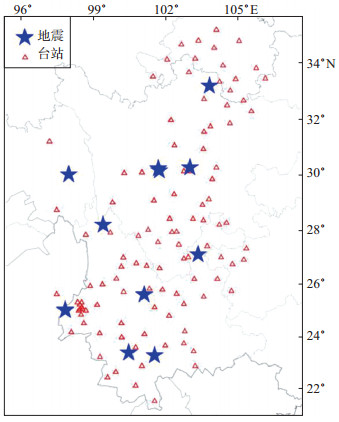图 1 本研究所使用台站和震中分布 Fig.1 Distribution of the stations and epicenters in this study
3 线性回归分析及Mew测定实例 3.1 回归分析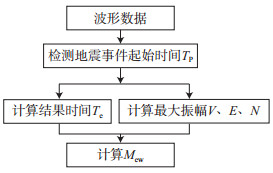图 2 自动测定Mew流程 Fig.2 The flow chart of the automatic determination of Mew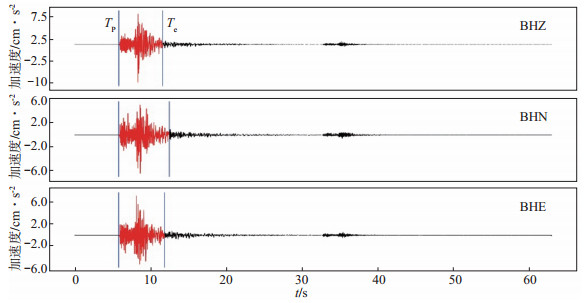图 3 YIM台站记录2018年9月8日云南墨江MW 5.7地震三分量波形 Fig.3 The three-component waveform of the MW 5.7 earthquake occurred on September 8, 2018, in Mojiang, Yunnan Province, recorded at YIM station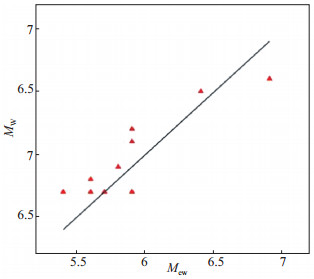图 4 12次地震Mew与MW的对应关系 Fig.4 The relationship between Mew and MW for 12 earthquakes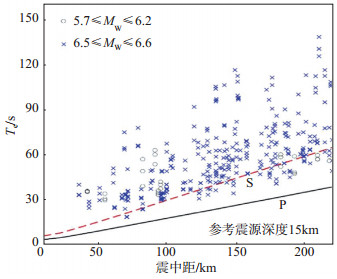图 5 145条强震动持续记录的结束时间分布 Fig.5 Distribution of the end time for 145 strong motion records used in this study

 $\log \sqrt {{E_{\rm{S}}}} = - 0.4442 + 0.8109{M_{\rm{W}}} - 0.002R - 0.4273\log R$ (6)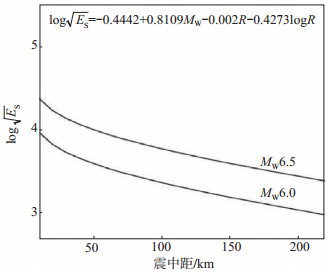图 6 MW = 6.0和6.5时的线性回归曲线 Fig.6 Regression curves for MW = 6.0 and 6.5

 ${M_{{\rm{eW}}}}{\rm{ = }}0.6166{E_{\rm{S}}} + 0.002R + 0.2569\log R + 0.5478$ (7)
3.2 Mew测定实例

4 结论与讨论

 陈晓佳, 薛兵. 2018. 近场P波频谱分布及震级确定方法[J]. 地震, 38(3): 123-133. DOI:10.3969/j.issn.1000-3274.2018.03.012 郭铁龙, 张雪梅, 邹立晔. 2017. STA/LTA-AIC算法对地震P波震相拾取稳定性影响[J]. 地震地磁观测与研究, 38(3): 13-17. DOI:10.3969/j.issn.1003-3246.2017.03.003 刘瑞丰, 陈运泰, 周公威, 等. 1999. 地震矩张量反演在地震快速反应中的应用[J]. 地震学报, 11061106(2): 115-122. DOI:10.3321/j.issn:0253-3782.1999.02.001 刘瑞丰, 陈运泰, 王丽艳. 2018. 新的震级国家标准的技术要点与主要特点[J]. 地震地磁观测与研究, 39(1): 1-11. 孙丽, 梁建宏. 2016. MWP震级测定方法在全球中强地震自动测定中的应用[J]. 地球物理学进展, 31(5): 2139-2144. 吴忠良, 陈运泰, 倪江川, 等. 1994. 近震源宽频带记录的地震矩张量反演[J]. 地震学报, 16(2): 141-152. 邹立晔, 黄志斌, 周静, 等. 2019. 2019年6月17日四川长宁M 6.0地震的快速测定与数据产品产出[J]. 中国地震, 35(3): 573-583. DOI:10.3969/j.issn.1001-4683.2019.03.016 Allen R M, Kanamori H. 2003. The potential for earthquake early warning in Southern California[J]. Science, 300(5620): 786-789. DOI:10.1126/science.1080912 Trnkoczy A.2002. Understanding and parameter setting of STA/LTA trigger algorithm[C]//IASPEI New Manual of Seismological Observatory Practice. Potsdam:Deutsches GeoForschungsZentrum, 2:119. Tsuboi S, Abe K, Takano K, et al. 1995. Rapid determination of MW from broadband P waveforms[J]. Bulletin of the Seismological Society of America, 85(2): 606-613. Wu Y M and Teng T L. 2004. Near real-time magnitude determination for large crustal earthquake[J]. Tectonophysics, 390(1/4): 205-216.